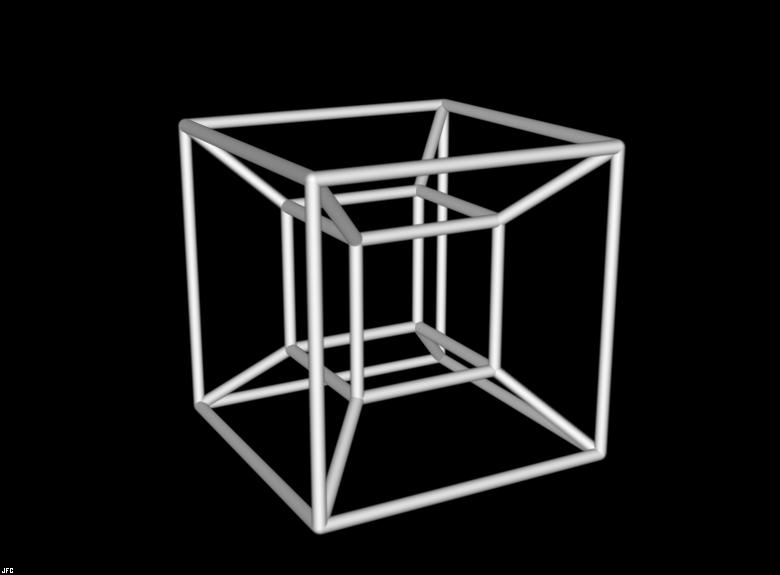A# tesseractA model of a projection of a (four-dimensional) tesseract in three-dimensions, analogous to a wireframe sketch a cube on a flat piece of paper.

A tesseract is the four-dimensional analogue of a cube, also known as a 4-space hypercube or an 8-cell. The name, possibly coined by Charles Hinton, comes from tessera, meaning four, and aktis, meaning ray, thus "four rays."

Just as a cube is obtained by "thickening" a square in the third dimension, which can be imagined as stacking infinitely many infinitely thin sheets of paper, a tesseract is a cube thickened in the fourth dimension. We can't imagine this because we can't think four dimensionally but it is possible to appreciate that just as perspectives of cubes can be drawn on a 2-d surface, so real cubes can serve as perspectives of tesseracts. A square drawn inside a larger square with the vertices connected by lines is one way to provide a perspective of a cube. Similarly, a hypercube is sometimes portrayed as a small cube within a larger cube with lines drawn from the vertices of the smaller cube to the vertices of the larger cube (see accompanying illustration). This kind of representation is a bit misleading, however, and reveals very little of the nature of a tesseract. It doesn't show, for instance, how a tesseract can be subdivided into smaller 4-d blocks in the same way that a cube can be divided into smaller cubes, or a square into smaller squares. A more useful way to think of a tesseract is as a folding, in the fourth dimension, of a 3-d net of eight cubes, just as a cube is a folding in the third dimension of a 2-d net of six squares. Start with a stack of four cubes, with four more cubes arranged in a cross around the second cube from the top. A tesseract is made by folding (in the fourth dimension) so that the top face of the cube at the top of the stack merges with the bottom face of the bottom cube, and so that the adjacent edges of the cubes in the cross join.

A tesseract is bounded by eight hyperplanes, each of which intersects it to form a cube. Two cubes, and so three squares, intersect at each edge. There are three cubes meeting at every vertex, the vertex polyhedron of which is a regular tetrahedron, leading to the Schläfi symbol {4,3,3}. The distance between opposite corners of a hypercube is twice the length of a side – much tidier than the corresponding values of √2 for a square and √3 for a cube.

 square cube tesseract vertices 4 8 16 edges 4 12 32 squares 1 6 24 cubes – 1 8

If a cube is hung from one of its vertices and sliced horizontally through its center, the result is a hexagon. What if the same is done to a tesseract? The slice will yield a 3-dimensional object – but what kind? The answer: an octahedron. By analogy with the slice of the 3-cube, the slice of the 4-cube must cut every "face." The number of faces of a 4-cube is eight and the only regular eight-sided solid is an octahedron.

Tesseracts turn up in both art and literature. Salvador Dali's Christus Hypercubus shows Christ being crucified on a tesseract. In Robert Heinlein's short story "And He Built a Crooked House" (1940), a house built as a three-dimensional projection of a tesseract collapses, or folds up, to become a real tesseract – with unusual consequences for the person trapped inside. The tesseract is also mentioned in Madeleine l'Engle's children's fantasy A Wrinkle In Time as a way of introducing the concept of higher dimensions.

### References

1. Hendricks, John R. "Black and White Vertices of a Hypercube." Journal of Recreational Mathematics, 11(4), 1978–1979.
2. Turney, P, "Unfolding the Tesseract." Journal of Recreational Mathematics, 17 (1): 1–16, 1984–85.